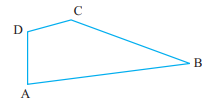# Name the angles in the given figure."

To do:

We have to name the angles in the given figure.

Solution:

The angles in the given figure are:

$\angle DAB, \angle ABC, \angle BCD$ and $\angle CDA$.